# Introduction to Equilibria

This is part of the HSC chemistry course under the topic Static and Dynamic Equilibrium

### HSC Chemistry Syllabus

• Model static and dynamic equilibrium and analyse the differences between open and closed systems (ACSCH079, ACSCH091)

### What is Equilibrium?

This section of the video will introduce the idea of open, closed, and insulated systems, and dynamic equilibrium

### Open and Closed Systems

• A system is an environment in which a reaction occurs. The system does not just concern itself with the chemical reactants involved but also the energy associated with the chemical reaction.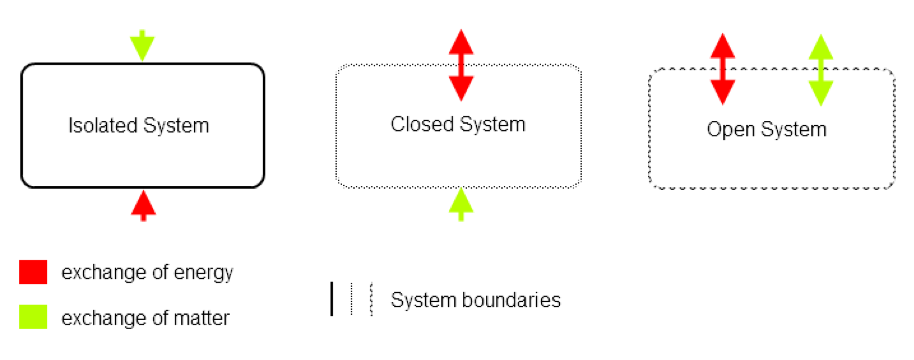• An open system is one where matter and energy are free to move in and out of it. A characteristic of an open system is the ability for the exchange of both matter (particles, molecules and compounds) and energy with outside of the system

Boiling water is a common example of an open system because the system which contains the boiling water; a kettle, freely allows water to move in and out of the kettle, and also allows heat to be dispersed outside.

• A closed system is one where the exchange of matter is prohibited but the exchange of energy is not.
Examples of closed systems are also quite common. E.g. A coffee cup with a tightly sealed lid. While matter (coffee) cannot move in and out of the cup, energy freely dissipates as the coffee cools down.

• Reversible reactions can only occur in closed systems. Products must remain in the system in order to be converted back into reactants.

Thermal Decomposition of Calcium Carbonate

$$CaCO_{3(s)} \rightarrow CO_{2(g)} + CaO_{(s)}$$

Thermal decomposition of CaCO3 goes to completion in an open system because carbon dioxide is no longer in contact with CaO.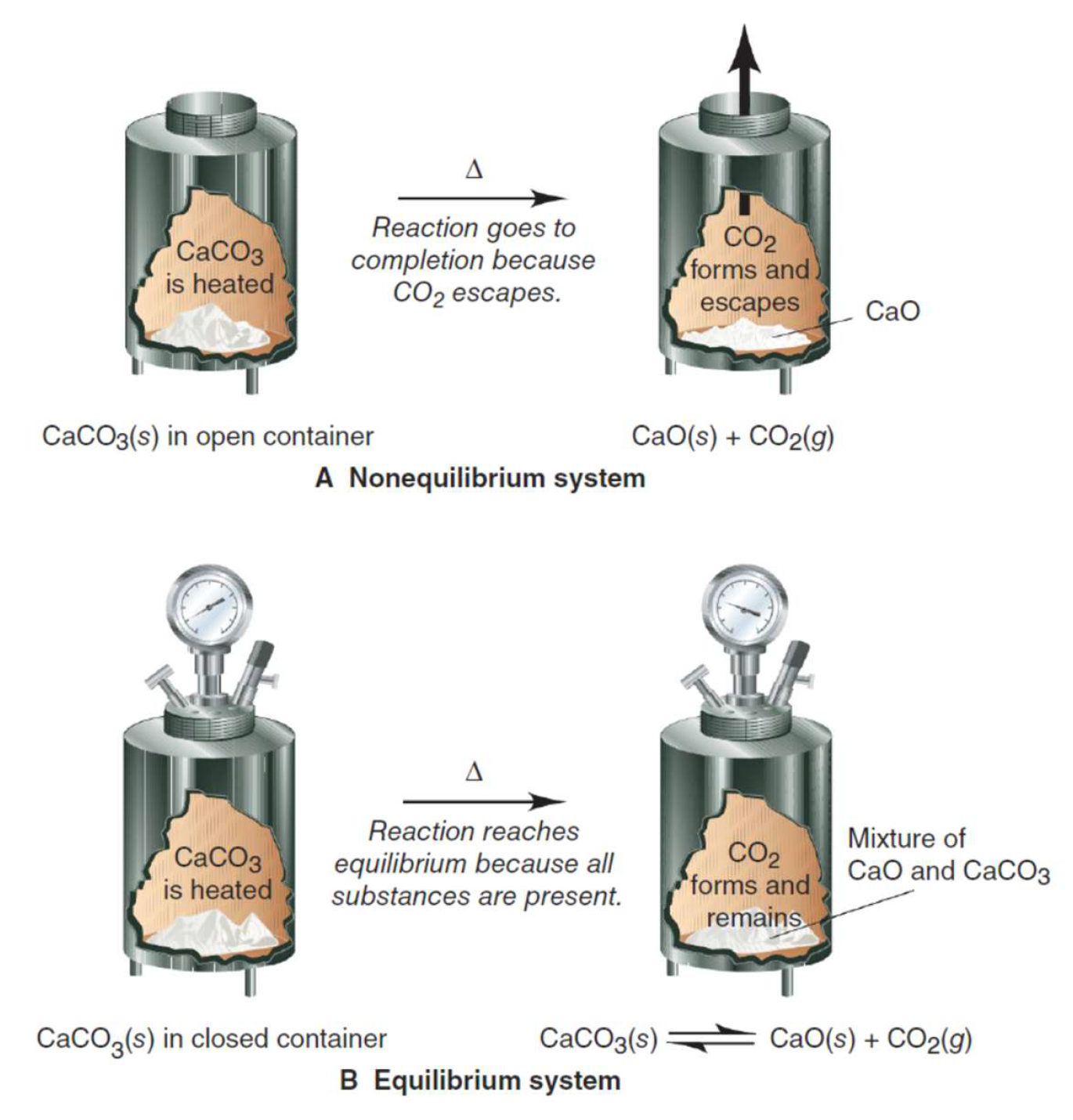Figure shows the thermal decomposition of calcium carbonate. This reaction is only reversible in a closed chemical system as products remain in contact with reactants.

$$CaCO_{3(s)} \rightleftharpoons CO_{2(g)} + CaO_{(s)}$$

In contrast, the reaction reaches equilibrium in a closed system when products and reactants remain together.

### Static vs Dynamic Equilibrium

What is a Static Equilibrium?
• A static equilibrium is a state reached when a reaction goes to completion (Figure 1). At this stage, the rates of forward and reverse reaction both equal zero.Figure 1: diagram illustrates an example of static equilibrium. All reactants (blue) are converted into products (yellow). The forward and reverse reaction rates are both zero.

• Static equilibrium can occur in two situations:
1. When all reagents or the limiting reagent have been consumed and converted into products.
1. When the activation energy of a reaction is too large to overcome under a particular setting e.g. temperature or pressure is too low. The movement between molecules/particles is too slow to overcome this energy.

• At static equilibrium, there are no dynamic forces acting on the reactants nor the products. As a result, at the point of equilibrium the reaction practically stops and there are no movements between reactants and products. Thus a reaction which has reached a static equilibrium is said to be irreversible.

What is a Dynamic Equilibrium?

• Reactants and products which are in dynamic equilibrium are acted upon by dynamic forces so there are movements between reactants and products. However, the rates of forward and reverse reaction are equal.Figure 2: diagram illustrates an example of dynamic equilibrium. Forward and reverse reaction rates are non-zero and equal. This results in no net gain or loss of reactants nor products. A chemical system at dynamic equilibrium seems to have no-visible reaction.

• In a closed system, any reversible reaction will reach a state of equilibrium. At this point, there is no macroscopic change as the overall concentrations of reactants and products stay the same.
• At a microscopic or molecular level, reactants and products are charging into one another at the same rate. A dynamic equilibrium exists in a reaction when rates of forward and backward reactions equal and are non-zero in value.
• The change in Gibbs free energy for both the forward and reverse reactions at equilibria is zero \text{(ΔG = 0}). This means the forward and reverse reactions are equally as spontaneous.

### Modelling a Dynamic Equilibrium

Suppose there are two identical containers, one initially filled with water and the other without water. A beaker is used to transfer the water between the two containers until the water levels become equal.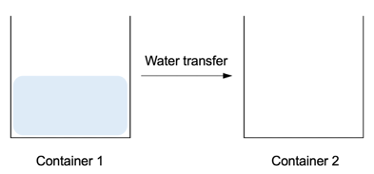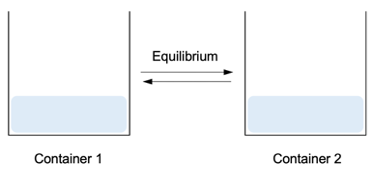• The model represents a closed system where the total amount of water remains constant in the two containers.
• The amount of water in each container represents the concentration of reactant and product.
• The water level represents the rate of forward and reverse reaction. When equilibrium is reached, the water levels are equal and remain unchanged.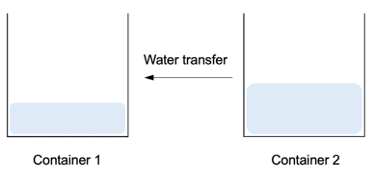• The transfer between containers represents the reversibility of the reaction. If too much water is transferred to the second container, some of the excess water can be transferred back to the first container. This procedure is repeated until water levels become equal.

• Limitation of this model: at equilibrium, the model does not show microscopic changes that happen between reactants and products.

### Practice Questions

Which one of the following chemical systems are reversible?

(i) Self-ionisation of water

(ii) Precipitation between ionic compounds in a beaker.

(iii) Acid-base neutralisation in a beaker.

(iv) Combustion of fuel e.g. octane

Solutions

(i) Self-ionisation of water (in any vessel) is an example of reversible reaction. While the vessel may not be completely sealed, the system is a closed one because water at room temperature (298 K) is unlikely to vaporise. Hence, the products of ionisation remain in the vessel and in contact with each other.(ii) Precipitation in a beaker is a closed chemical system. However, in most cases the reaction is irreversible because the reverse reaction is thermodynamically unfavoured. An example of this is the precipitation between sodium chloride and silver nitrate.

$$NaCl_{(aq)} + AgNO_{3(aq)} \rightarrow NaNO_{3(aq)} + AgCl_{(s)}$$

(iii) Acid-base neutralisation in a beaker (school laboratory setting) is usually not reversible because it occurs in an open system. The water is more likely to vaporise given that neutralisation is an exothermic reaction. This means water, being one of the products, can leave the system.

In addition to acid-base reactions, acid and carbonate reactions are also unlikely to be reversible because carbon dioxide can freely leave the beaker.

(iv) Combustion of fuel is an irreversible reaction due to a few reasons. First of all, if it occurs in an open system, carbon dioxide and water can freely leave the system.

$$2C_8H_{18(l)} + 25O_{2(g)} \rightarrow 18H_2O_{(g)} + 16CO_{2(g)}$$

The reverse reaction of combustion is also extremely thermodynamically unfavourable as it would be endothermic and entail a decrease in entropy (more on this in the next section).

Next Section: Non-equilibrium Systems